# Year 2 Maths Printable Worksheets

Use our colourful year 2 maths worksheets to help your child master new maths skills! Our maths worksheets cover all the core objectives including problem-solving, shape and space, mental maths, number bonds, and measuring and recording results using tables. We also cover topics such as money, fractions, greater than/less than, ordering and comparing, and more.

Filters

### Least amount of coins

Year 2 maths - Least amount of coins worksheet. This is a primary resource for key stage 1 on money. The child needs to work out the least amount of coins needed for a certain amount of money. Having physical coins in front of the child help them with this process.

Age: 6 - 7
1
2
3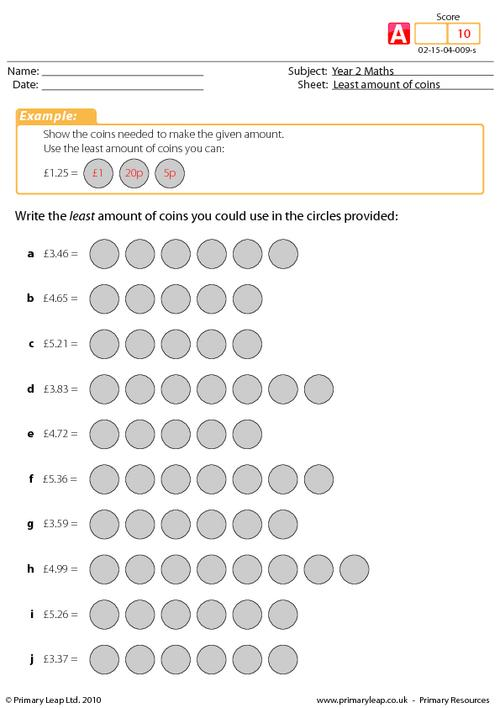### Least amount of coins

Year 2 maths - Least amount of coins worksheet. This is a primary resource for key stage 1 on money. The child needs to work out the least amount of coins needed for a certain amount of money. Having physical coins in front of the child help them with this process.

Age: 6 - 7
1
2
3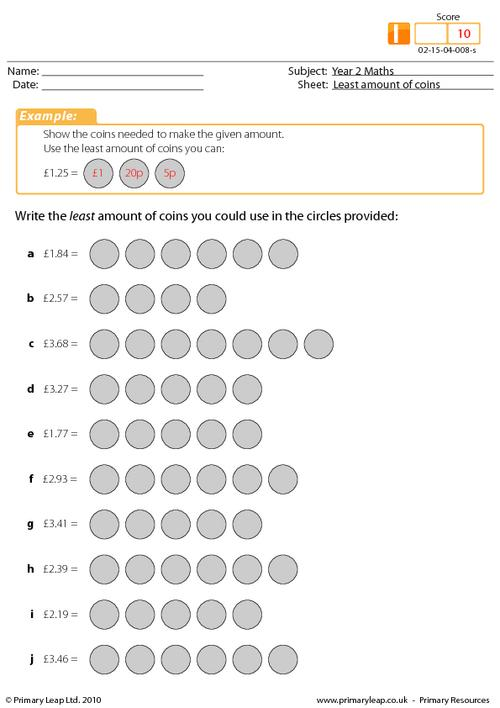### Least amount of coins

Year 2 maths - Least amount of coins worksheet. This is a primary resource for key stage 1 on money. The child needs to work out the least amount of coins needed for a certain amount of money. Having physical coins in front of the child help them with this process.

Age: 6 - 7
1
2
3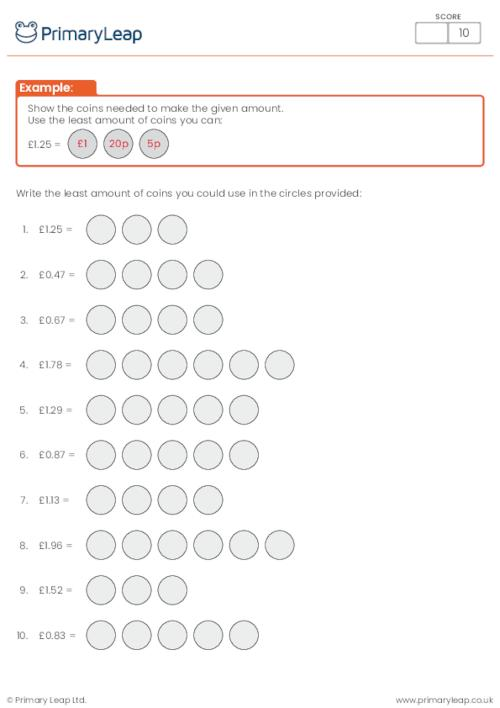### Giving change

Year 2 maths - Giving change worksheet. This activity asks pupils how much change they will get from 50p, 1 pound and 2 pounds after spending x amount.

Age: 6 - 7
1
2
3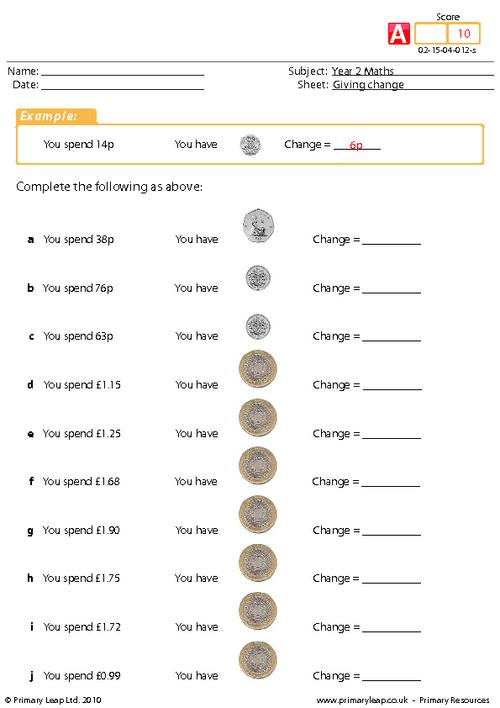### Giving change

Year 2 maths - Giving change worksheet. This activity asks pupils how much change they will get from 50p and 1 pound after spending x amount.

Age: 6 - 7
1
2
3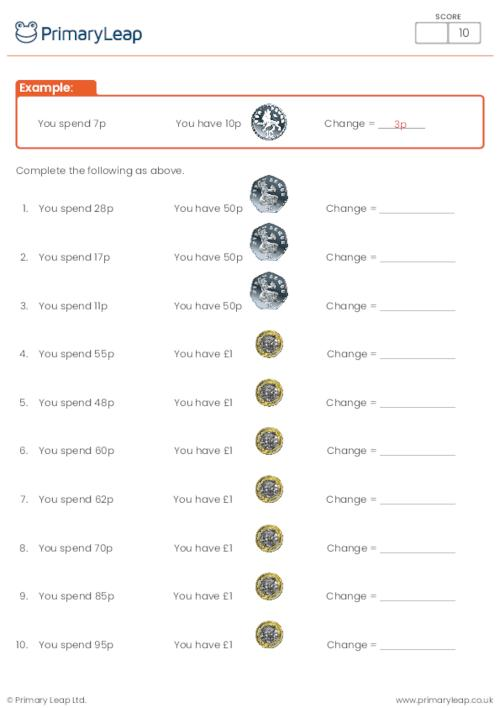### Giving change

Year 2 maths - Giving change worksheet. This activity asks pupils how much change they will get from 50p after spending x amount.

Age: 6 - 7
1
2
3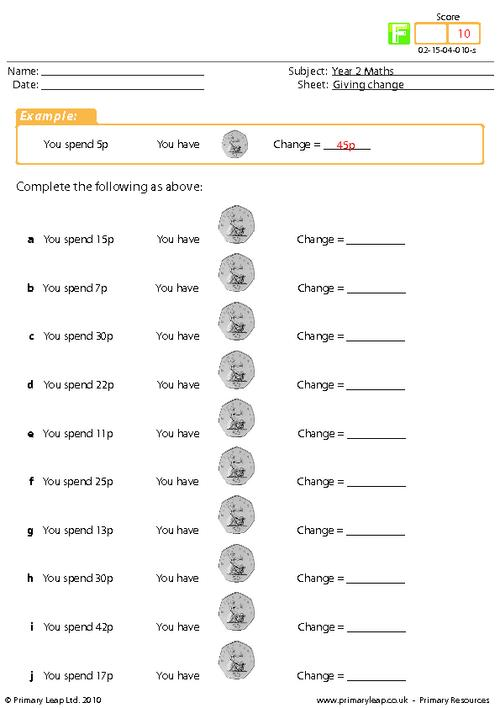### Money subtraction

Year 2 maths - Subtraction money worksheet. This activity will help students work out and practice how to subtract sums of money.

Age: 6 - 7
1
2
3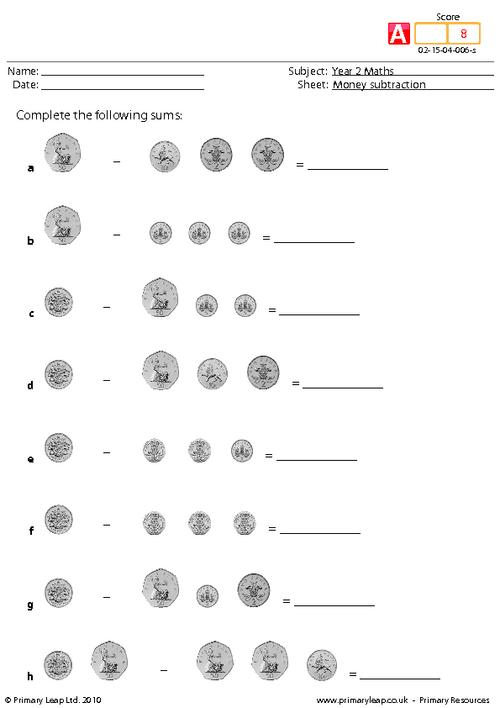### Money subtraction

Year 2 maths - Subtraction money worksheet. This activity will help students work out and practice how to subtract sums of money.

Age: 6 - 7
1
2
3### Money subtraction

Year 2 maths - Subtraction money worksheet. This activity will help students work out and practice how to subtract sums of money.

Age: 6 - 7
1
2
3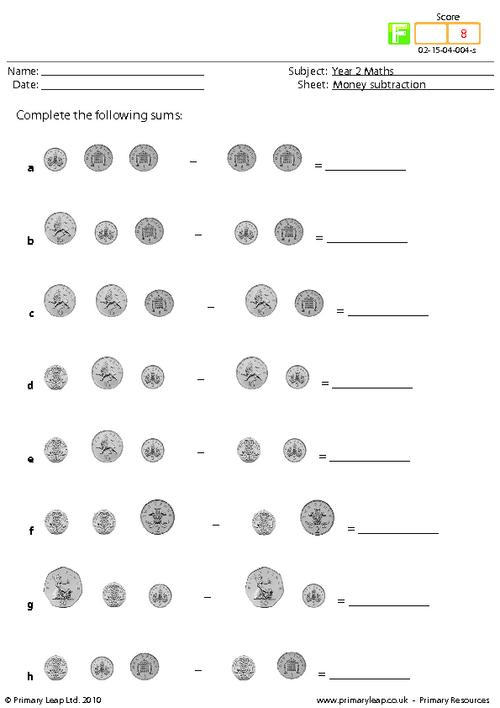### Counting coins

Year 2 maths - Money worksheet. This page asks pupils to add up the coins and give the total amount. This activity introduces pupils to recognising different coins and adding them correctly which is a skill that children will need throughout their lives.

Age: 6 - 7
1
2
3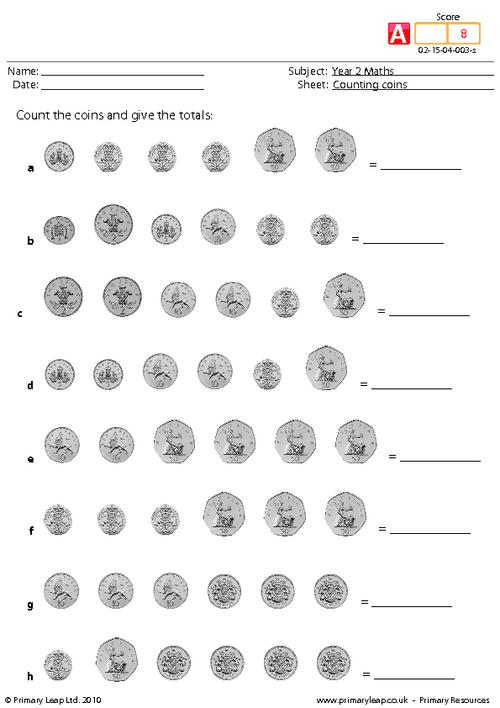### Counting coins

Year 2 maths - Money worksheet. This page asks pupils to add up the coins and give the total amount. This activity introduces pupils to recognising different coins and adding them correctly which is a skill that children will need throughout their lives.

Age: 6 - 7
1
2
3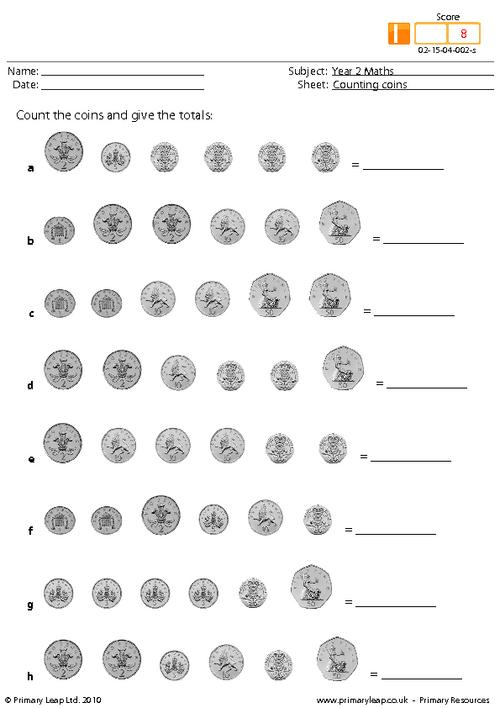### Counting coins

Year 2 maths - Money worksheet. This page asks pupils to add up the coins and give the total amount. This activity introduces pupils to recognising different coins and adding them correctly which is a skill that children will need throughout their lives.

Age: 6 - 7
1
2
3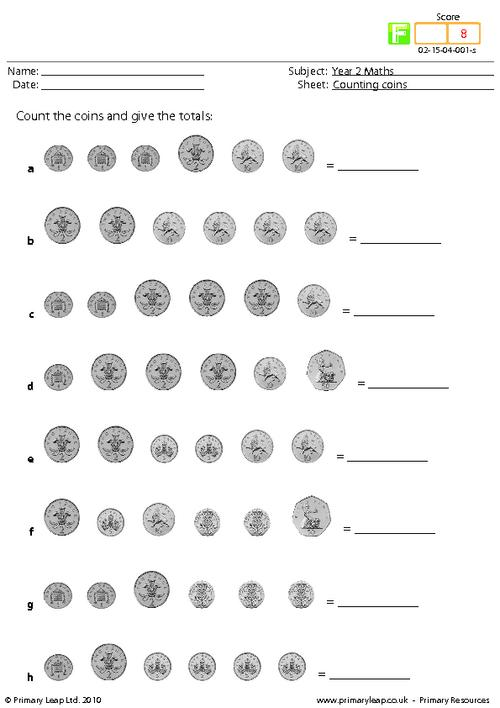of 1Help your child find the joy of learning year 2 maths with our engaging maths activities. We have hundreds of year 2 maths worksheets to boost your child's confidence. Our maths worksheets will help your child learn how to count in steps of 2, 3, 5 and 10, learn number bonds to 20, add and subtract one-digit and two-digit numbers, find fractions of shapes, and more.Learn

One of the best things about printable worksheets is that they make learning fun and easy. Explore our educational resources based on Year 2 Maths and keep your children learning through these fun and engaging exercises.Progress

Our Year 2 Maths printable worksheets are designed for progressive learning. Our engaging content enables a child to learn at their own speed and gain confidence quickly. When we help a child believe in themselves, we empower them for life.Improve

Printable worksheets are a great way to enhance creativity and improve a child’s knowledge. Help your child learn more about Year 2 Maths with this great selection of worksheets designed to help children succeed.